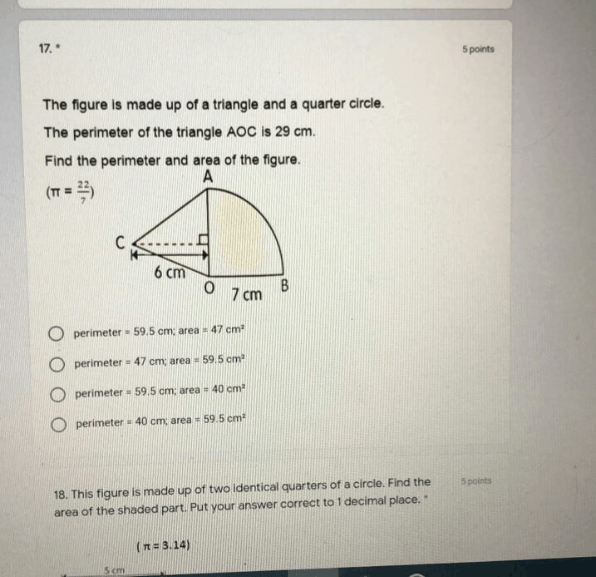# QuestionHi…can someone help me. The answer of perimeter is 40 or 47? Thanks advance

Since AO and OB are the radii of the quarter circle,

AO = OB = 7 cm

Area of trangle AOC = ½ x 7 x 6 = 21 cm2

As for the perimeter, we cannot find out the length of AC or OC individually.

However, we know AC, OC and AO have a combined perimeter of 29 cm. We also know the length of AO = 7 cm.

So, the combined length of AC and OC = 29 – 7 = 22 cm

The only length left to find out is the curved line.

¼ x 2 x πx 7 = 11 cm

Perimeter of figure = 11 + 7 + 22 = 40 cm

0 Replies 2 Likes ✔Accepted Answer